Courses

# Test: Stresses in Beams Level - 2

## 20 Questions MCQ Test Strength of Materials (SOM) | Test: Stresses in Beams Level - 2

Description
This mock test of Test: Stresses in Beams Level - 2 for Mechanical Engineering helps you for every Mechanical Engineering entrance exam. This contains 20 Multiple Choice Questions for Mechanical Engineering Test: Stresses in Beams Level - 2 (mcq) to study with solutions a complete question bank. The solved questions answers in this Test: Stresses in Beams Level - 2 quiz give you a good mix of easy questions and tough questions. Mechanical Engineering students definitely take this Test: Stresses in Beams Level - 2 exercise for a better result in the exam. You can find other Test: Stresses in Beams Level - 2 extra questions, long questions & short questions for Mechanical Engineering on EduRev as well by searching above.
QUESTION: 1

### Two beams a square of side ‘a’ and one with rectangular sides ‘a’ in width and 2a in depth are compared. The ratio of moment of inertia of square to rectangular section is

Solution: Moment of inertia of square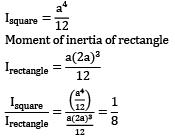QUESTION: 2

### A beam cross-section is used in two different orientations as shown in the figure given below.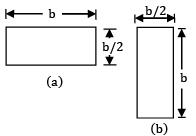Solution: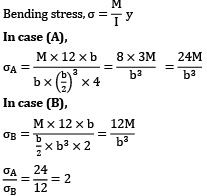σA = 2σB

QUESTION: 3

### The ratio of the curvature of the 3 loose beams. (b × d) placed one over the other to an integral beam (b × nd) is

Solution: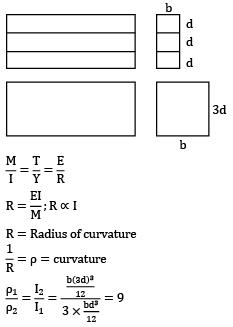QUESTION: 4

The maximum compressive stress at the top of a beam was 1600kg/cm2 and the corresponding tensile stress at its bottom was 800 kg/cm2. If the depth of the beam was 15 cm the neutral axis from the top will be

Solution: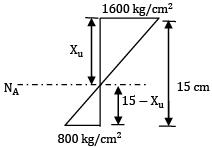Stress diagram By similar triangle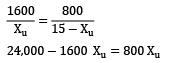Xu = 10 cm

QUESTION: 5

The width of the strongest beam of rectangular section that can be cut out of a cylindrical log of wood whose diameter is 30 cm would be (in cm)

Solution: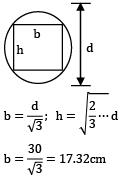QUESTION: 6

A cantilever of constant depth carries a uniformly distributed load on the whole span. To make the maximum stress at all sections the same, the breadth of the section at a distance ‘x’ from the free end should proportional to

Solution: At a distance ‘x’ from free end,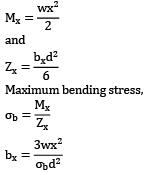∴ The breadth is proportional to x2 .

QUESTION: 7

A beam of T-section has I = 1000 cm4 and depth 10 cm. Flange of the section is in tension. If the maximum tensile stress is two times the maximum compressive stress, what is section modulus in compression?

Solution: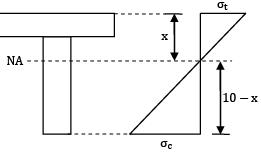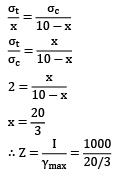= 150 cm3

QUESTION: 8

For the configuration of loading shown in the given figure, the stress in fiber AB is given by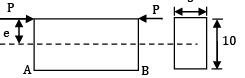Solution: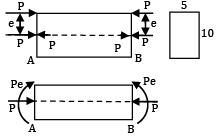σAB = σbending (T) + σaxial(C)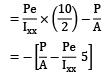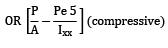QUESTION: 9

A beam having rectangular cross section 200mm wide and 400mm deep is simply supported over a span of 5m. It is carrying a concentrated load of 10 kN at the centre of the span. The maximum bending stress developed at quarter span of the beam is

Solution: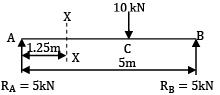Moment at quarter span, M = 5 × 1.25 = 6.25 kN­m

Maximum bending stress,

M = fZ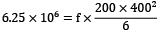f = 1.172 N/mm2

QUESTION: 10

Two beams of cross-section circular and square have the same length, same allowable bending stress and the same moment of resistance. The weight of the beam with circular section is K times that of the square section, where ‘K’ is

Solution: Zcircle = Zsquare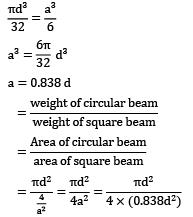K = 1.118

QUESTION: 11

For a rectangular section, keeping breadth ‘’b’’ constant, the depth ‘’d’’ for uniform strength beam will have relation with bending moment ‘’M’’ as

Solution: The strength of section is uniform

M = σb × Z

M = σb × bd2 / 6

M ∝ b

M ∝ d2

d ∝ √M

QUESTION: 12

If a beam is cut in halves horizontally and the two halves are laid side by side, it can in comparison to the original beam carry

Solution: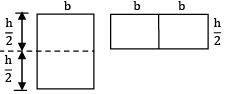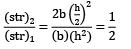QUESTION: 13

A hollow beam of square section with external dimensions of 50.0 mm and thickness 5.0 mm can sustain a stress of 100 MPa. Its capacity in flexure is

Solution: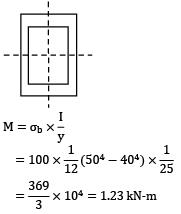QUESTION: 14

A square beam laid flat is then rotated in such a way that one of its diagonal becomes horizontal. How is its moment capacity affected?

Solution: Moment capacity of a beam is given by MR = σy × Z

For a particular beam σy is constant

∴ MR ∝ Z

When beam is laid flat, then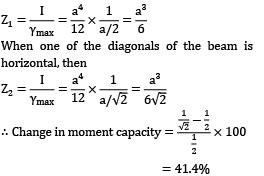∴ Moment capacity increases by 41.4%.

QUESTION: 15

A T-section beam is simply supported and subjected to a uniform distributed load over its whole span. Maximum longitudinal stress in the beam occurs at

Solution: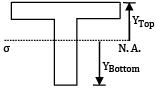YBottom > YTop

Since the bottom fiber is farthest from the neutral axis. It will experience maximum longitudinal stress.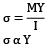Y is the distance of fiber from the neutral axis.

QUESTION: 16

The ratio of flexibility of ‘n’ loose beams (b × d) placed one over the other to the flexibility of one integral beam (nb × d) is

Solution: Flexibility of beam = 1 / stiffness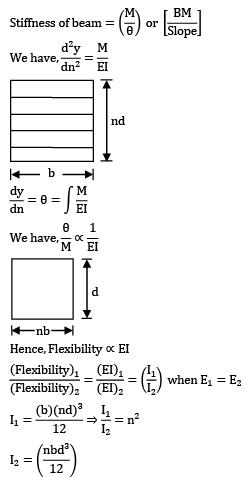Hence, Flexibility ⍺EIQUESTION: 17

A solid circular cross-section cantilever beam of diameter ϕ = 100 mm carries a shear force of 10 kN at the free end. The maximum shear stress is

Solution: d = 100 mm V = 10 kN = 104N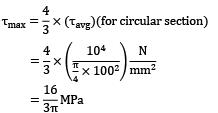QUESTION: 18

A wooden beam of rectangular cross section 100mm × 200mm is formed by gluing two identical beams of square cross section. If the safe shear stress of the glue is 3 N⁄mm2, the safe shear strength

Solution: Maximum shear stress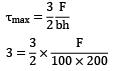F = 40 × 103N

= 40 kN

QUESTION: 19

The given figure (all dimensions are in mm) shows an I-section of the beam.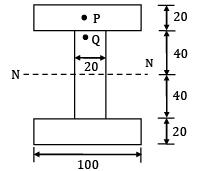Solution: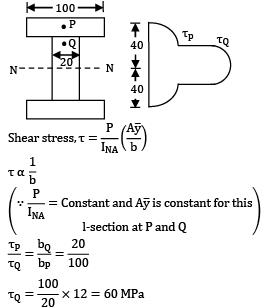QUESTION: 20

If a beam of rectangular cross-section is subject to a vertical shear force S, then how much shear force will be carried by the upper one third of the section?

Solution: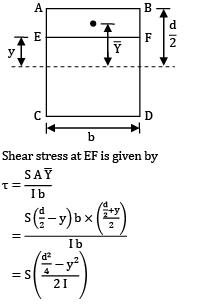Shear stress at EF is given by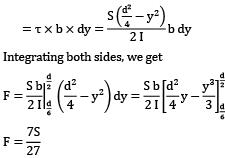Shear force carried by elementary strip of thickness ‘dy’

dF = τ × !rea of elementary strip